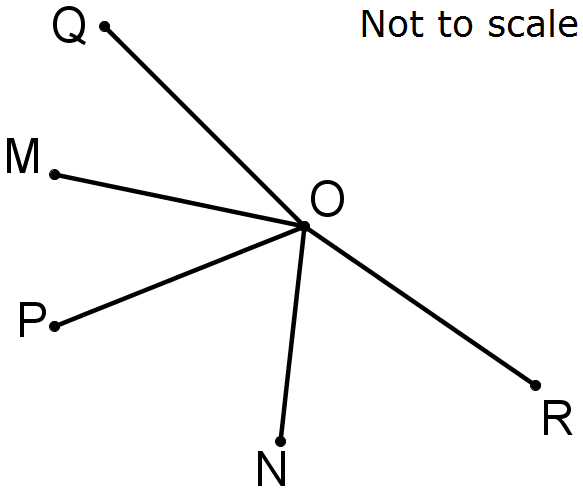You may also likeOn Time

On a clock the three hands - the second, minute and hour hands - are on the same axis. How often in a 24 hour day will the second hand be parallel to either of the two other hands?Estimating Angles

How good are you at estimating angles?LOGO Challenge 1 - Star Square

Can you use LOGO to create this star pattern made from squares. Only basic LOGO knowledge needed.

Angular Reflection

Age 11 to 14 ShortChallenge LevelIn the diagram, $\angle MON = 130^{\circ}$. The reflection of $OP$ in $OM$ is $OQ$ and the reflection of $OP$ in $ON$ is $OR$.

What is the size of $\angle QOR$?

If you liked this problem, here is an NRICH task which challenges you to use similar mathematical ideas.
This problem is taken from the UKMT Mathematical Challenges.
You can find more short problems, arranged by curriculum topic, in our short problems collection.